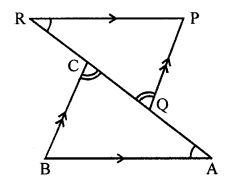## ML Aggarwal Class 7 Solutions for ICSE Maths Chapter 12 Congruence of Triangles Objective Type Questions

Mental Maths

Question 1.
Fill in the blanks:
(i) Two line segments are congruent if ……….
(ii) Among two congruent angles, one has a measure of 63°; the measure of the other angle is ……….
(iii) When we write ∠A = ∠B, we actually mean ………
(iv) The side included between ∠M and ∠N of ∆MNP is ……….
(v) The side QR of ∆PQR is included between angles ……….
(vi) If two triangles ABC and PQR are congruent under the correspondence A ↔ R, B ↔ P and C ↔ Q, then in symbolic form it can be written as ∆ABC = ………
(vii) If ∆DEF = ∆SRT, then the correspondence between vertices is ……….
Solution:
(i) Two line segments are congruent if they are of the same length.
(ii) Among two congruent angles, one has a measure of 63°;
the measure of the other angle is 63°.
(iii) When we write ∠A = ∠B, we actually mean m∠A = m∠B.
(iv) The side included between ∠M and ∠N of ∆MNP is MN.
(v) The side QR of ∆PQR is included between angles ∠Q and ∠R.
(vi) If two triangles ABC and PQR are congruent
under the correspondence A ↔ R, B ↔ P and C ↔ Q,
then in symbolic form it can be written as ∆ABC = ∆RPQ.
(vii) If ∆DEF = ∆SRT, then the correspondence between vertices is
D ↔ S, E ↔ R and F ↔ T.

Question 2.
State whether the following statements are true (T) or false (F):
(i) All circles are congruent.
(ii) Circles having equal radii are congruent.
(iii) Two congruent triangles have equal areas and equal perimeters.
(iv) Two triangles having equal areas are congruent.
(v) Two squares having equal areas are congruent.
(vi) Two rectangles having equal areas are congruent.
(vii) All acute angles are congruent.
(vii)All right angles are congruent.
(ix) Two figures are congruent if they have the same shape.
(x) A two rupee coin is congruent to a five rupee coin.
(xi) All equilateral triangles are congruent.
(xii) Two equilateral triangles having equal perimeters are congruent.
(xii) If two legs of one right triangle are equal to two legs of another right angle triangle, then the two triangles are congruent by SAS rule.
(xiv) If three angles of two triangles are equal, then triangles are congruent.
(xv) If two sides and one angle of one triangle are equal to two sides and one angle of another triangle, then the triangle are congruent.
Solution:
(i) All circles are congruent. (False)
Correct:
As if all circles have equal radii otherwise not.
(ii) Circles having equal radii are congruent. (True)
(iii) Two congruent triangles have equal areas
and equal perimeters. (True)
(iv) Two triangles having equal areas are congruent. (False)
Correct:
As they may have different sides and angles.
(v) Two squares having equal areas are congruent. (True)
(vi) Two rectangles having equal areas are congruent. (False)
Correct:
As their side can be different.
(vii) All acute angles are congruent. (False)
Correct:
As acute angles have different measures.
(viii) All right angles are congruent. (True)
(ix) Two figures are congruent if they have the same shape. (False)
Correct:
As the same shapes have different measures.
(x) A two rupee coin is congruent to a five rupee coin. (False)
Correct:
As they have different size.
(xi) All equilateral triangles are congruent. (False)
Correct:
As they have different sides in length.
(xii) Two equilateral triangles having equal perimeters are congruent. (True)
(xiii) If two legs of one right triangle are equal to
two legs of another right angle triangle,
then the two triangles are congruent by SAS rule. (True)
(xiv) If three angles of two triangles are equal,
then triangles are congruent. (False)
Correct:
They can be similar to each other.
(xv) If two sides and one angle of one triangle are equal to two sides
and one angle of another triangle, then the triangle is congruent. (False)
Correct:
If the angles are included, they can be congruent.

Multiple Choice Questions

Choose the correct answer from the given four options (3 to 14):
Question 3.
Which one of the following is not a standard criterion of congruency of two triangles?
(a) SSS
(b) SSA
(c) SAS
(d) ASA
Solution:
The axiom SSA is not a standard criterion
of congruency of triangles. (b)

Question 4.
If ∆ABC = ∆PQR and ∠CAB = 65°, then ∠RPQ is
(a) 65°
(b) 75°
(c) 90°
(d) 115°
Solution:
∆ABC = ∆PQR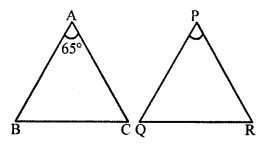∠CAB = 65°
∠RPQ = 65° (corresponding angles) (a)

Question 5.
If ∆ABC = ∆EFD, then the correct statement is
(a) ∠A = ∠D
(b) ∠A = ∠F
(c) ∠A = ∠E
(d) ∠B = ∠E
Solution:
∆ABC = ∆EFD
Then ∠A = ∠E (c)Question 6.
If ∆ABC = ∆PQR, then the correct statement is
(a) AB = QR
(b) AB = PR
(c) BC = PR
(d) AC = PR
Solution:
∆ABC = ∆PQR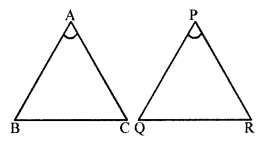Then AB = PQ
AC = PR (d)

Question 7.
If ∠D = ∠P, ∠E = ∠Q and DE = PQ, then ∆DEF = ∆PQR, by the congruence rule
(a) SAS
(b) ASA
(c) SSS
(d) RHS
Solution:
In ∆DEF = ∆PQR
∠D = ∠P, ∠E = ∠Q
DE = PQ
∆DEF = ∆PQR (ASA axiom) (b)

Question 8.
In ∆ABC and ∆PQR, BC = QR and ∠C = ∠R. To establish ∆ABC = ∆PQR by SAS congruence rule, the additional information required is
(a) AC = PR
(b) AB = PR
(c) CA = PQ
(d) AB = PQ
Solution:
If ∆ABC = ∆PQR by SAS
BC = QR and ∠C = ∠R, then AC = PR (a)

Question 9.
In the given figure, the lengths of the sides of two triangles are given. The correct statement is
(a) ∆ABC = ∆PQR
(b) ∆ABC = ∆QRP
(c) ∆ABC = ∆QPR
(d) ∆ABC = ∆RPQ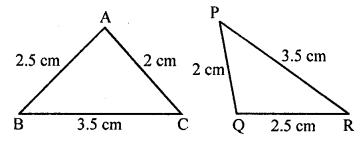Solution:
Correct statement is ∆ABC = ∆QRP. (b)

Question 10.
In the given figure, M is the mid-point of both AC and BD. Then
(a) ∠1 = ∠2
(b) ∠1 = ∠4
(c) ∠2 = ∠4
(d) ∠1 = ∠3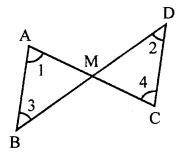Solution:
In the given figure,
M is mid-point of AC and BD both then ∠1 = ∠4. (b)

Question 11.
In the given figure, ∆PQR = ∆STU. What is the length of TU?
(a) 5 cm
(b) 6 cm
(c) 7 cm
(d) cannot be determined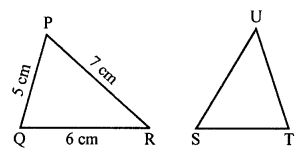Solution:
In the given figure,
∆PQR = ∆STU
TU = QR = 6 cm (b)

Question 12.
In the given figure, ∆ABC and ∆DBC are on the same base BC. If AB = DC and AC = DB, then which of the following statement is correct?
(a) ∆ABC = ∆DBC
(b) ∆ABC = ∆CBD
(c) ∆ABC = ∆DCB
(d) ∆ABC = ∆BCDSolution:
In the given figure,
AB = DC, AC = DB
Then, ∆ABC = ∆DCB (c)

Question 13.
The two triangles shown in the given figure are:
(a) congruent by AAS rule
(b) congruent by ASA rule
(c) congruent by SAS rule
(d) not congruent.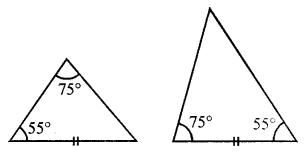Solution:
In the given two triangles are not congruent.
In first triangle, AAS are given while in second ASA are given. (d)

Question 14.
In .the given figure, ∆ABC = ∆PQR. The values of x and y are:
(a) x = 63, y = 35
(b) x = 77, y = 35
(c) x = 35, y = 77
(d) x = 63, y = 40Solution:
In the given figure,
∆ABC = ∆PQR
∠A = ∠P and ∠B = ∠Q
Now x – 7 = 70°
⇒ x = 70° + 7 = 77°
and 2y + 5 = 75
⇒ 2y = 75° – 5 = 70°
⇒ y = 35°
x = 77°, y = 35° (b)

Higher Order Thinking Skills (HOTS)

Question 1.
If all the three altitudes of a triangle are equal, then prove that it is an equilateral triangle.
Solution:
Given: In ∆ABC,
AD, BE and CF are altitudes of the triangle
and AD = BE = CF.To prove: ∆ABC is an equilateral.
Proof: In ∆ABD and ∆CFB
∠D = ∠F (Each = 90°)
∠B = ∠B (Common)
∆ABD = ∆CFB (AAS criterion)
AB = BC …….(i)
∠C = ∠C (Common)
∠E = ∠D (Each = 90°)
BC = AC ………(ii)
From (i) and (ii)
AB = BC = AC
∆ABC is an equilateral triangle.

Question 2.
In the given fig., if BA || RP, QP || BC and AQ = CR, then prove that ∆ABC = ∆RPQ.Solution:
In the given figure, BA || RP
QP || BC and AQ = CR
To prove : ∆ABC = ∆RPQ
Proof: AQ = CR